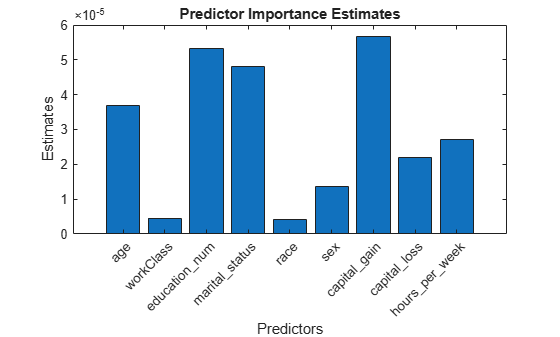predictorImportance

Estimates of predictor importance for classification tree

Syntax

imp = predictorImportance(tree)

Description

imp = predictorImportance(tree) computes estimates of predictor importance for tree by summing changes in the risk due to splits on every predictor and dividing the sum by the number of branch nodes.

Input Arguments

 tree A classification tree created by fitctree, or by the compact method.

Output Arguments

 imp A row vector with the same number of elements as the number of predictors (columns) in tree.X. The entries are the estimates of predictor importance, with 0 representing the smallest possible importance.

Examples

expand all

Grow a classification tree.

Mdl = fitctree(meas,species);

Compute predictor importance estimates for all predictor variables.

imp = predictorImportance(Mdl)
imp = 1×4

0         0    0.0907    0.0682

The first two elements of imp are zero. Therefore, the first two predictors do not enter into Mdl calculations for classifying irises.

Estimates of predictor importance do not depend on the order of predictors if you use surrogate splits, but do depend on the order if you do not use surrogate splits.

Permute the order of the data columns in the previous example, grow another classification tree, and then compute predictor importance estimates.

measPerm  = meas(:,[4 1 3 2]);
MdlPerm = fitctree(measPerm,species);
impPerm = predictorImportance(MdlPerm)
impPerm = 1×4

0.1515         0    0.0074         0

The estimates of predictor importance are not a permutation of imp.

Grow a classification tree. Specify usage of surrogate splits.

Mdl = fitctree(meas,species,'Surrogate','on');

Compute predictor importance estimates for all predictor variables.

imp = predictorImportance(Mdl)
imp = 1×4

0.0791    0.0374    0.1530    0.1529

All predictors have some importance. The first two predictors are less important than the final two.

Permute the order of the data columns in the previous example, grow another classification tree specifying usage of surrogate splits, and then compute predictor importance estimates.

measPerm  = meas(:,[4 1 3 2]);
MdlPerm = fitctree(measPerm,species,'Surrogate','on');
impPerm = predictorImportance(MdlPerm)
impPerm = 1×4

0.1529    0.0791    0.1530    0.0374

The estimates of predictor importance are a permutation of imp.

Load the census1994 data set. Consider a model that predicts a person's salary category given their age, working class, education level, martial status, race, sex, capital gain and loss, and number of working hours per week.

'sex','capital_gain','capital_loss','hours_per_week','salary'});

Display the number of categories represented in the categorical variables using summary.

summary(X)
Variables:

age: 32561x1 double

Values:

Min          17
Median       37
Max          90

workClass: 32561x1 categorical

Values:

Federal-gov            960
Local-gov             2093
Never-worked             7
Private              22696
Self-emp-inc          1116
Self-emp-not-inc      2541
State-gov             1298
Without-pay             14
NumMissing            1836

education_num: 32561x1 double

Values:

Min           1
Median       10
Max          16

marital_status: 32561x1 categorical

Values:

Divorced                   4443
Married-AF-spouse            23
Married-civ-spouse        14976
Married-spouse-absent       418
Never-married             10683
Separated                  1025
Widowed                     993

race: 32561x1 categorical

Values:

Amer-Indian-Eskimo       311
Asian-Pac-Islander      1039
Black                   3124
Other                    271
White                  27816

sex: 32561x1 categorical

Values:

Female     10771
Male       21790

capital_gain: 32561x1 double

Values:

Min            0
Median         0
Max        99999

capital_loss: 32561x1 double

Values:

Min            0
Median         0
Max         4356

hours_per_week: 32561x1 double

Values:

Min           1
Median       40
Max          99

salary: 32561x1 categorical

Values:

<=50K     24720
>50K       7841

Because there are few categories represented in the categorical variables compared to levels in the continuous variables, the standard CART, predictor-splitting algorithm prefers splitting a continuous predictor over the categorical variables.

Train a classification tree using the entire data set. To grow unbiased trees, specify usage of the curvature test for splitting predictors. Because there are missing observations in the data, specify usage of surrogate splits.

Mdl = fitctree(X,'salary','PredictorSelection','curvature',...
'Surrogate','on');

Estimate predictor importance values by summing changes in the risk due to splits on every predictor and dividing the sum by the number of branch nodes. Compare the estimates using a bar graph.

imp = predictorImportance(Mdl);

figure;
bar(imp);
title('Predictor Importance Estimates');
ylabel('Estimates');
xlabel('Predictors');
h = gca;
h.XTickLabel = Mdl.PredictorNames;
h.XTickLabelRotation = 45;
h.TickLabelInterpreter = 'none';In this case, capital_gain is the most important predictor, followed by education_num.